# An equilateral

An equilateral triangle with a side of 10 m represents a wooden platform standing on a lawn. A goat is tied to a corner with a 15 m rope. What is the maximum amount of grazing area available to the goat?

S =  663.5571 m2

### Step-by-step explanation: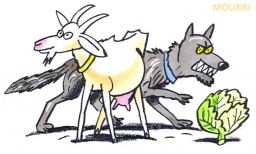Did you find an error or inaccuracy? Feel free to write us. Thank you!Tips to related online calculators

#### You need to know the following knowledge to solve this word math problem:

We encourage you to watch this tutorial video on this math problem:

## Related math problems and questions:

• Equilateral triangle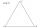Find the area of an equilateral triangle with a side of 15 cm.
• Goat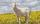Meadow is a circle with a radius r = 19 m. How long must a rope tie a goat to the pin on the perimeter of the meadow to allow the goat to eat half of the meadow?
• Circular lawnAround a circular lawn area is 2 m wide sidewalk. The outer edge of the sidewalk is curb whose width is 2 m. Curbstone and the inner side of the sidewalk together form a concentric circles. Calculate the area of the circular lawn and the result round to 1
• LawnsBefore a sports hall is two equally large rectangular lawns, each measuring 40 m and 12 m. Maintenance of 10m² lawn costs 45 CZK yearly. On each lawn is a circular flowerbed with a diameter of 8 meters. How much money is needed each year to take on lawn c
• Parcelparcel has a rectangular shape of a trapezoid with bases 12 m and 10 m and a height 8 m. On parcel was built object with a footprint an isosceles triangle shape with side 4 m and height three-quarters of a meter. What is the area of unbuild parcel?
• Goat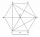The fenced flower bed has the shape of a regular hexagon. The tops are formed by fence posts. The fence around the flowerbed measures 60 m. A goat is tied to one of the pillars from the outside and grazes on the surrounding meadow (the goat should not ent
• An angleAn angle x is opposite side AB which is 10, and side AC is 15 which is hypotenuse side in triangle ABC. Calculate angle x.
• Roof tilesThe roof has a trapezoidal shape with bases of 15 m and 10 m, height of roof is 4 meters. How many tiles will need if on 1 m2 should be used 8 tiles?
• Goat and circlesWhat is the radius of a circle centered on the other circle and the intersection of the two circles is equal to half the area of the first circle? This task is the mathematical expression of the role of agriculture. The farmer has circular land on which g
• Seagull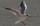Artificially created island in the shape of a circle with a radius of 50 m is overgrown with grass. The only exception is a landing area for helicopters in the shape of a rectangle measuring 15 m and 8 m. What is the probability that the flying seagull (w
• Triangular prism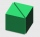Calculate the surface of a triangular prism with the base of an equilateral triangle with a side length of 7.5 cm and a corresponding height of 6.5 cm. Prism height is 15cm.
• Equilateral triangle v3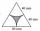Calculate the content of the colored gray part. Equilateral triangle has side length 8 cm. Arc centers are the vertices of a triangle.
• Water levelHow high is the water in the swimming pool with dimensions of 37m in length and 15m in width, if an inlet valve is opened for 10 hours flowing 12 liters of water per second?
• The gardenThe garden has the shape of an isosceles trapezoid whose bases are 64 m and 24 m long, the height is 25 m. On what area of the garden is it possible to grow vegetables if a fifth of the area is occupied by a cottage, lawn and path?Calculate the radius of the quadrant, which area is equal to area of circle with radius r = 15 cm.The roof of the tower has the shape of a regular quadrangular pyramid, the base edge of which is 11 m long and the side wall of the animal with the base an angle of 57°. Calculate how much roofing we need to cover the entire roof, if we count on 15% wasteThe carpenters made wooden mold on a concrete pedestal in the shape of a cube with an edge 2 meters long. What is the area in which the concrete touches wooden molds? (No lid or bottom)